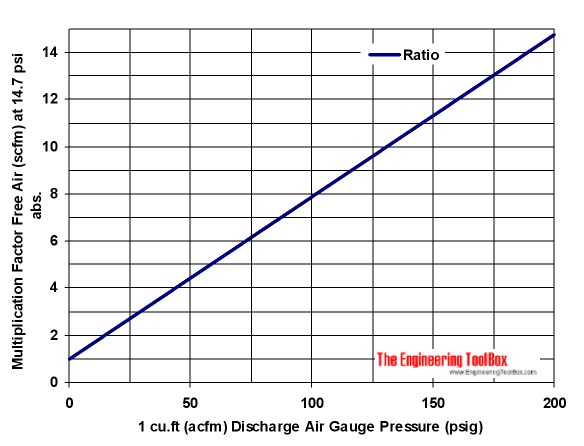Engineering ToolBox - Resources, Tools and Basic Information for Engineering and Design of Technical Applications!

# Compressed Air vs. Free Air - Compression Ratio

## The ratio of compressed air pressure to free air pressure.

Free air is air at ambient conditions at a specific location where

• ambient temperature
• moisture content
• barometric pressure

are stated.

### Compression Ratio

Compression Ratio is based on the Ideal Gas Law and is the ratio between Discharge Pressure Absolute and Suction Pressure Absolute.

CR = pd / ps                                (1)

where

CR = compression ratio

pd = discharge absolute pressure (bar abs, psia)

ps  = suction absolute pressure (bar abs, psia)

The compression ratio of free air - to compressed air, is indicated in the diagram below.

### Compression Ratio - Pressure (psi)• 1 psi = 6.9 kPa = 0.069 bar
• 1 ncfm = 0.5 nl/s

#### Converting Compressed Air Volume Flow to Free Air Volume Flow

A compressed air volume flow can be converted to a free air volume flow by using the equation

qF = CR qC                            (2)

where

qF = free air flow (m3/s, cfm)

qC = compressed air flow (m3/s, cfm)

#### Example - Converting Compressed Air Volume Flow to Free Air Volume Flow

A compressed air volume flow of 10 acfm (actual cfm) at 100 psig must be multiplied with Compression Ratio approximately 8 to estimate the volume of free air at atmospheric pressure.

qF = 8 (10 acfm)

= 80 scfm  (standard cfm)

### Compression Ratio - Pressure (bar)#### Example - Converting Free Air Volume Flow to Compressed Air Volume Flow

A free air volume flow of 1 m3/s is compressed to 10 bar (gauge) and must be divided with Compression Ratio approximately 11 to estimate the volume of compressed air.

qC = (1 m3/s) / 11

= 0.091 m3/s

## Engineering ToolBox - SketchUp Extension - Online 3D modeling!

Add standard and customized parametric components - like flange beams, lumbers, piping, stairs and more - to your Sketchup model with the Engineering ToolBox - SketchUp Extension - enabled for use with the amazing, fun and free SketchUp Make and SketchUp Pro .Add the Engineering ToolBox extension to your SketchUp from the SketchUp Pro Sketchup Extension Warehouse!

Translate

## Privacy

We don't collect information from our users. Only emails and answers are saved in our archive. Cookies are only used in the browser to improve user experience.

Some of our calculators and applications let you save application data to your local computer. These applications will - due to browser restrictions - send data between your browser and our server. We don't save this data.

## Citation

• Engineering ToolBox, (2008). Compressed Air vs. Free Air - Compression Ratio. [online] Available at: https://www.engineeringtoolbox.com/free-compressed-air-d_1190.html [Accessed Day Mo. Year].

Modify access date.

. .

#### Scientific Online Calculator6 24